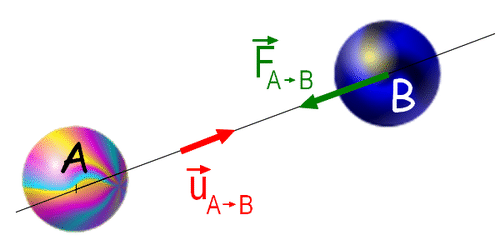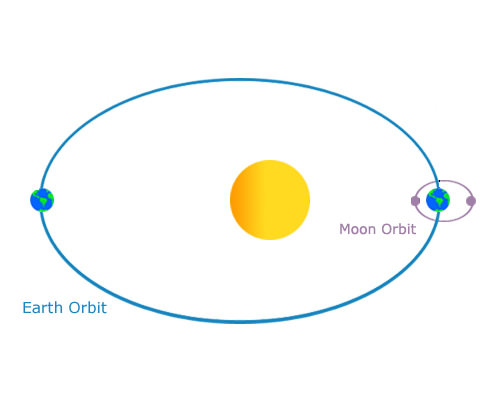# 22.8: Gravity in the Solar System

•• Contributed by CK12
• CK12Have you ever heard the old adage, "What goes up must come down"?

Every moment of every day is a field trip to gravity. Gravity is everywhere! You have a gravitational attraction to your dog. You have one to your pencil. You even have one to your school principal! These gravitational attractions are very small compared with the most important one you have. This is your gravitational attraction to Earth. It's what keeps you from floating off into space. Gravity holds our planet together. Gravity keeps Earth orbiting the Sun. We wouldn't be here without gravity.

## The Role of Gravity

All objects in the universe have an attraction to each other. This attraction is known as gravity (Figure below). The strength of the force of gravity depends on two things. One is the mass of the objects. The other is the distance between the objects. As an object's mass increases, the attraction increases. As the distance between the objects increases, the attraction decreases.The strength of the force of gravity between objects A and B depends on the mass of the objects and the distance (u) between them.

Isaac Newton first described gravity as the force that causes objects to fall to the ground. Gravity is also the force that keeps the Moon circling Earth. Gravity keeps Earth circling the Sun. Without gravity, these objects would fly off into space (Figure below).The Moon orbits the Earth, and the Earth-Moon system orbits the Sun.

Earth's gravity pulls any object on or near Earth toward the planet's center.

## Summary

• All objects have a gravitational attraction to each other. This is called gravity.
• The attraction is proportional to the mass of the objects. The attraction is inversely proportional to the distance between the objects.
• Gravity keeps the Moon orbiting Earth. Gravity keeps the planets orbiting the Sun.

## Review

1. For which object is the force of gravity greatest: Earth, Moon, or Sun? Why?
2. Imagine that the Moon and the Sun are the same distance from Earth. Which one would Earth be gravitationally attracted to?
3. What is gravity?

## Explore More

Use the resource below to answer the questions that follow.

1. Who was Isaac Newton?
2. What did Newton discover?
3. How did Newton make his discovery?